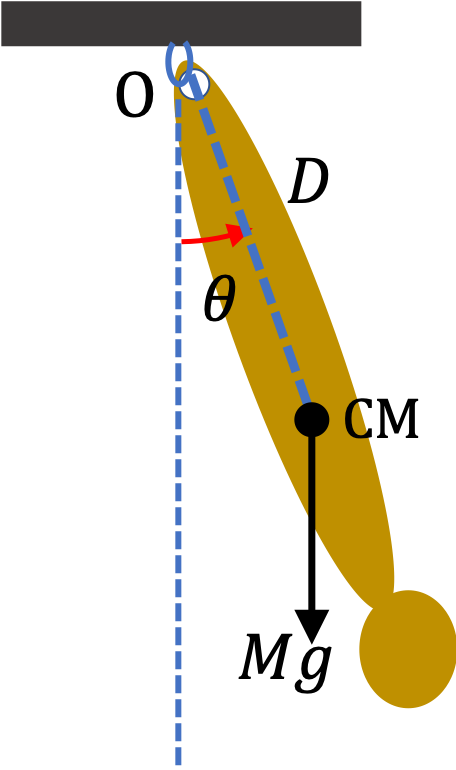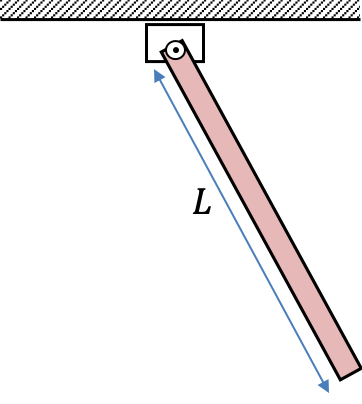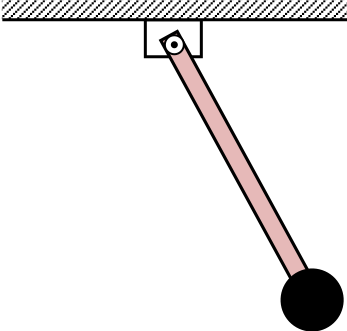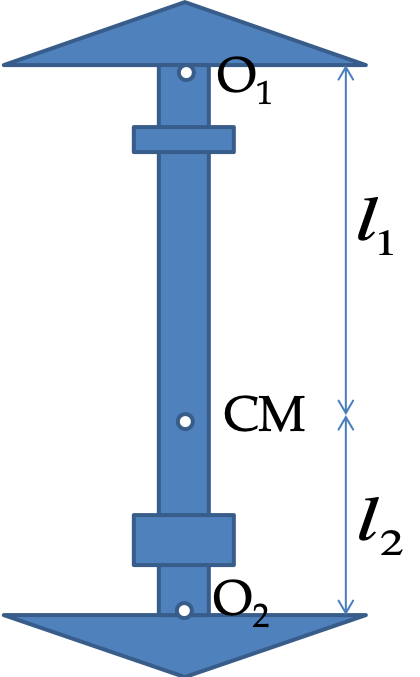## Section13.4Physical Pendulum

A rigid body hung from a post swings just like a pendulum. Such oscillating bodies are called physical pendulums. We want to find the frequency of small oscillations.

Almost anything can be a physical pendulum. An illustration in shown in the figure. The torque responsible for the oscillations comes from the force of gravity on the body. To calculate the torque of weight we place the weight vector at the center of mass of the body but for the moment of inertial we use the actual shape of the object.

Let $M$ be the mass of the body swinging about a suspension point. Let $D$ be the distance from the suspension point to the center of mass. Only weight has non-zero lever arm about the axis through the suspension point.The torque of the weight when the body's CM is at an angle $\theta$ with respect to the vertical.

\begin{equation*} \tau_\text{net} = - M g D \theta, \end{equation*}

where the negative sign refers to the clockwise sense of rotation. Let $I$ be moment of inertia about the axis and $\alpha$ the angular acceleration. Then, the equation of motion

\begin{equation} I\alpha = - MgD\theta.\tag{13.4.1} \end{equation}

Rewriting this as

\begin{equation*} \alpha = - \dfrac{MgD}{I}\,\theta. \end{equation*}

Comparing this to the equation of motion of a simple harmonic motion, $a_x = -\omega^2 x \text{,}$ and of simple pendulum in small angle approximation, $\alpha = -\omega^2 \theta\text{,}$ we find that the angular frequency of the physical pendulum is

\begin{equation} \omega = \sqrt{ \dfrac{MgD}{I} }. \tag{13.4.2} \end{equation}

This gives frequency $f$ to be

\begin{equation} f = \dfrac{\omega}{2\pi} = \dfrac{1}{2\pi}\,\sqrt{ \dfrac{MgD}{I} },\tag{13.4.3} \end{equation}

and the period $T\text{,}$

\begin{equation} T = \dfrac{1}{f} = {2\pi}\,\sqrt{ \dfrac{I}{MgD} }.\tag{13.4.4} \end{equation}

A rod of mass $10\text{ kg}$ and length $1.5\text{ m}$ is pivoted at one end. What is the frequency (in $\text{Hz}$) of small oscillations of the rod?Hint

Treat rod as a physical pendulum.

$0.5\text{ Hz}\text{.}$

Solution

The moment of inertia of the rod about the pivot point is

\begin{equation*} I = \dfrac{1}{3}ML^2. \end{equation*}

The distance from pivot to the center of mass of the rod is

\begin{equation*} D = \dfrac{L}{2}. \end{equation*}

Therefore, frequency of small oscillations will be

\begin{align*} f \amp = \dfrac{1}{2\pi}\,\sqrt{ \dfrac{MgD}{I} } \\ \amp = \dfrac{1}{2\pi}\,\sqrt{ \dfrac{3g}{2L} } = \dfrac{1}{2\pi}\,\sqrt{ \dfrac{3\times 9.81}{2\times 1.5} } = 0.5\text{ Hz}. \end{align*}

A physical pendulum has pivot at one of a rod and a spherical ball at the other end. The rod has mass $m$ and length $l$ and ball has mass $M$ and radius $R\text{.}$ Assume the center of the ball to be at a distance $l+R$ from the pivot point. What is the frequency of oscillation?

Numerical values: $m=10\text{ kg}\text{,}$ $l = 1.5\text{ m}\text{,}$ $M=20\text{ kg}\text{,}$ $R=0.25\text{ m}$Hint

Find $D$ from CM formula and $I$ by adding up $I$ of parts.

$0.40\text{ Hz}\text{.}$

Solution

The center of mass of the rod plus ball system will be at distance $D$ from the pivit, given by

\begin{equation*} D = \dfrac{1}{m+M}\left( \dfrac{1}{2}m l + M(l + R) \right). \end{equation*}

The moment of inertia of the rod plus ball system about the pivot point is

\begin{equation*} I = \dfrac{1}{3}m l^2 + \left( \dfrac{2}{5} M R^2 + M(l+R)^2 \right). \end{equation*}

We put these in the formula for frequency to get the answer.

\begin{align*} f \amp = \dfrac{1}{2\pi}\,\sqrt{ \dfrac{(m+M) g D}{I} } \end{align*}

Numerical values:

\begin{align*} (m+M) D \amp = \left( \dfrac{1}{2}m l + M(l + R) \right) \\ \amp = \dfrac{1}{2}\times 10\times 1.5 + 20(1.5 + .25)=7.5+35=42.5 \\ I \amp = \dfrac{1}{3}m l ^2 + \left( \dfrac{2}{5} M R^2 + M(l+R)^2 \right) \\ \amp = \dfrac{1}{3}\times 10 \times 1.5^2 + \left( \dfrac{2}{5} \times 20\times .25^2 + 20(1.5+.25)^2 \right) \\ \amp = 7.5 + \left( 0.5 + 61.25 \right) = 69.25 \end{align*}

Therefore,

\begin{align*} f \amp = \dfrac{1}{2\pi}\,\sqrt{ \dfrac{42.5\times 9.81}{69.25} } = 0.40\text{ Hz}. \end{align*}

To determine the value of acceleration due to gravity from a physical pendulum one needs to determine the time period and the moment of inertia of the pendulum. However, the moment of inertia is usually difficult to determine experimentally. The Kater's pendulum is a special physical pendulum that removes the necessity of knowing the moment of inertia by using the periods from two suspension points.

The Kater's pendulum consists of a long metallic rod with a slide-able weight on the rod. The pendulum has two knife edges at two ends that are used to suspend the pendulum from a support with a hole over which the knife edge rests. By adjusting the pivot points and the mass distribution over the rod, it is possible to obtain a configuration such that the time periods of oscillations about the two suspension points $O_1$ and $O_2$ are equal.Let $k$ be radius of gyration of the pendulum, $l_1$ and $l_2$ the distances from the center of gravity to the suspensions $\text{O}_1$ and $\text{O}_2$ respectively. Let $T_1$ and $T_2$ be the time periods of oscillations when Kater's pendulum is hung from knife edges $O_1$ and $O_2$ respectively.

(a) Find the time periods $T_1$ and $T_2$ in terms of $l_1,\ l_2,\ k\text{,}$ and $g \text{.}$

(b) Prove that when the time periods are equal, the period is given by $T = 2\pi \sqrt{\frac{L}{g}}\text{,}$ where $L=l_1+l_2\text{,}$ the distance between the two knife edges.

Hint

(a) Use $I_1 = I_\text{cm} + Ml_1^2$ with $I_\text{cm}=Mk^2\text{.}$ (b) Set $T_1=T_2\text{,}$ solve for $k^2\text{,}$ and use that $k^2\text{.}$

(a) $T_i = 2\pi\,\sqrt{ \dfrac{k^2 +l_i^2}{gl_i} }\text{,}$ $i=1,2\text{,}$ (b) Answer already given.

Solution 1 (a)

(a) From the definition of radius of gyration, we have the following for moment of inertia about an axis through the center of mass

\begin{equation*} I_\text{cm} = Mk^2. \end{equation*}

Using the parallel axis theorem, the moment of inertia from suspension point $\text{O}_1$ will be

\begin{equation*} I_1 = I_\text{cm} + Ml_1^2 = M \left(k^2 +l_1^2\right). \end{equation*}

Similarly,

\begin{equation*} I_2 = I_\text{cm} + Ml_2^2 = M \left(k^2 +l_2^2\right). \end{equation*}

The time period of a physical pendulum is

\begin{equation*} T = 2\pi\,\sqrt{ \dfrac{I}{Mgl} }, \end{equation*}

where $l$ is the distance from suspension to the center of mass. Therefore, the two periods are

\begin{align*} T_1 \amp = 2\pi\,\sqrt{ \dfrac{k^2 +l_1^2}{gl_1} }, \\ T_2 \amp = 2\pi\,\sqrt{ \dfrac{k^2 +l_2^2}{gl_2} }. \end{align*}
Solution 2 (b)

(b) With $T_1=T_2$ we have $T_1^2 = T_2^2\text{.}$ Upon simplifincation we get

\begin{equation*} l_2\left( k^2 + l_1^2\right) = l_1\left( k^2 + l_2^2\right), \end{equation*}

which can be solved for $k^2\text{,}$

\begin{equation*} k^2 = l_1l_2. \end{equation*}

Putting this in either $T_1$ or $T_2$ gives

\begin{equation*} T_1 = 2\pi\sqrt{ \dfrac{l_1l_2 + l_1^2}{gl_1} } = 2\pi\sqrt{ \dfrac{l_1 + l_2}{g} } = 2\pi\sqrt{ \dfrac{L}{g} }. \end{equation*}

We get the same result from $T_2\text{.}$